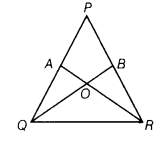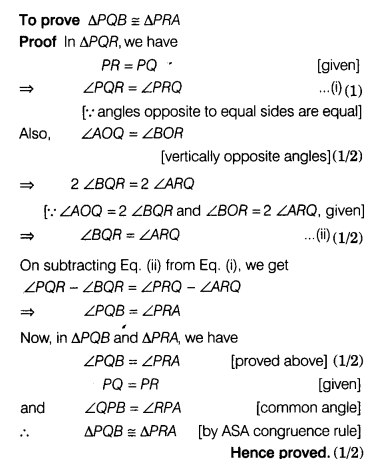# Given figure, if PQ = PR,∠AOQ = 2∠BQR and∠BOR = 2∠ARQ,prove that ∆PQB=∆PRA

In the given figure, if PQ = PR, ∠AOQ = 2∠BQR and ∠BOR = 2∠ARQ,
then prove that ∆PQB = ∆PRA.Given A ∆PQR in which PQ = PR, ∠AOQ = 2∠BQR and ∠BOR =2∠ARQ